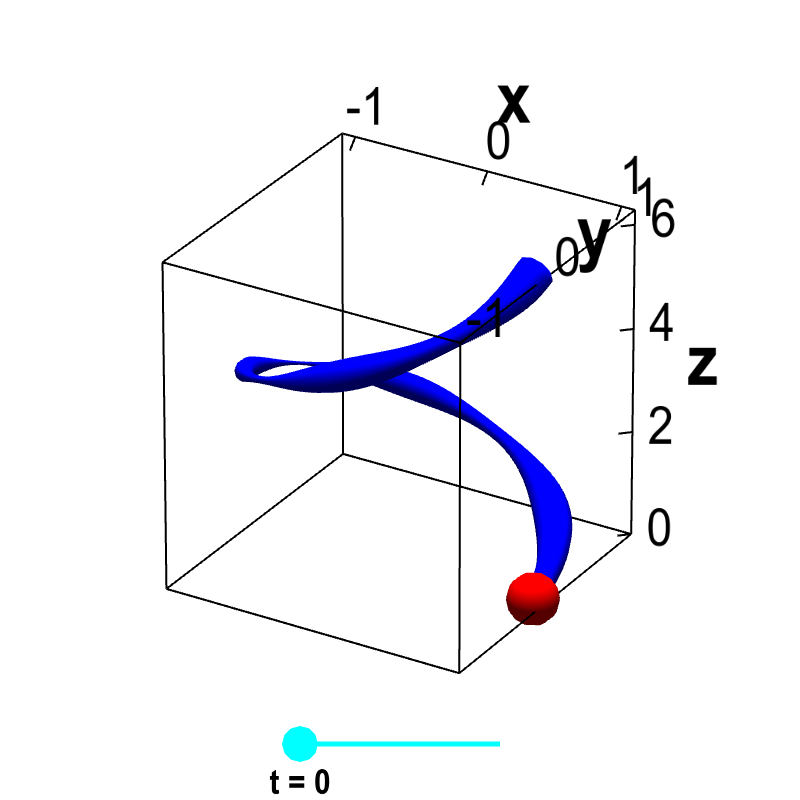# Math Insight

### Applet: Helix with variable densityThe function $\dllp(t) = (\cos t, \sin t, t)$, for $0 \le t \le 2\pi$ parametrizes a helix. The blue curve representing the helix is drawn with a variable width, representing the varying density $\dlsi(x,y,z)$ of the helix at each point $(x,y,z)=\dllp(t)$ along its length. By dragging the cyan point representing $t$ on the slider, one can move the red point $\dllp(t)$ along the helix.